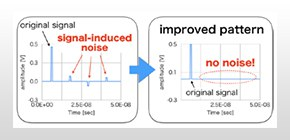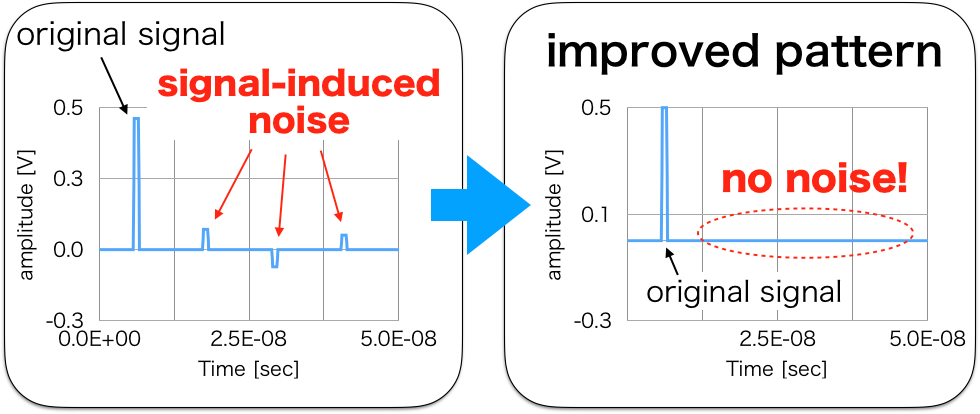## Computational algorithm to reduce electromagnetic noise in electronic circuits developed

### Osaka University researchers develop a computational algorithm for describing electromagnetic noise in electric circuits using a method based on electromagnetic studies and circuit theory

Jan 30, 2019Engineering

In order to design noiseless electromagnetic (EM) devices, it is necessary to clarify the mechanism behind EM noise and theoretical calculations and computer simulations are performed for prediction assessment of devices. Two researchers at Osaka University developed an algorithm for numerical calculation of EM noise (interference) in electric circuits.

EM noise is a problem that has proven to be difficult to solve. Caused by interference from transmission lines and connecting parts, various approaches have been taken to reduce it, such as adding filters and/or passive devices to circuits or using the symmetry of the configuration.

The algorithm developed in this study is for computer simulation of electric circuits in which transmission lines are connected with lumped element models. Usually, the solution of problems along a transmission line is performed using partial differential equations, while the solution of problems in a lumped constant circuit uses ordinary differential equations. In order to connect these two different differential equations and solve these problems, the pair of researchers, Prof. Masayuki Abe and Prof. Hiroshi Toki, introduced the incidence matrix found in circuit theory and time domain impedance, the latter of which is a new concept.

Previously, this solution required a method to replace lumped constant circuits with transmission lines, but this new method does not require such a replacement, allowing for more practical calculations. The results of this research were published in Scientific Reports .

Based on the results of calculations using this algorithm, the two researchers demonstrated that EM noise could be reduced by using the symmetric 3-line configuration of the circuit. Their calculation method is for one-dimensional multi-conductor transmission lines, but they have already developed a calculation algorithm in two- and three-dimensional multi-conductor transmission lines (patent pending) as well, making it possible to advance its applied research.

This calculation method can also be developed into a calculation method for the retarding effects of EM noise (and signals), which are difficult to calculate via conventional computer simulations. Currently, the researchers are developing a computational algorithm for calculating these retarding effects. In addition to analysis of time and frequency domains of EM noise, this algorithm will be used for various applications, such as generation of heat by noise, metamaterials, and antenna analysis.

“Eventually, we aim to develop an 'EM noise-less infrastructure.' In addition to improving device performance, we’d like to realize a society in which people can use high value-added equipment, such as equipment with ultra-low power consumption and ultra-low waste heat,” says Prof. Abe. “Specifically, we will theoretically clarify a noiseless structure of electronic circuits and demonstrate that drastic reduction of EM noise can lead to a breakthrough that allows for low power consumption.”

Prof. Toki says, “Our goal is to use our method to develop advanced technology into general purpose technology and establish guidelines for developing the concept of noiseless electronic devices into projects with both social and economic impact.”Figure: Examples of the research result.
A pulse signal induces a series of pulses on the transmission line (left). Improvement of the transmission line arrangement and lumped circuit connection provides “noise-free” signal transmission (right).
(credit: Osaka University)

The article, “Theoretical Study of Lumped Parameter Circuits and Multiconductor Transmission Lines for Time-Domain Analysis of Electromagnetic Noise” was published in Scientific Reports at DOI: https://doi.org/10.1038/s41598-018-36383-3 .